## Blog Moved

This website completely moved to new domain. For latest content, visit www.programmingposts.com

## 21 Jul 2012

### C Program to Add Two Numbers Using Function

Write a c program to add two numbers / integers using function..

Note: The same program can be used for adding floating numbers, just we have
to change the function return type,and all variables to float type and
for large numbers declare as long..

PROGRAM:

#include<stdio.h>
#include<conio.h>
int sum(int,int);   /*declaring prototype of function*/
void main()
{
int a,b,c;
printf("\n Enter the two numbers : ");
scanf("%d  %d",&a,&b);    /* taking two numbers as input*/
c = sum(a,b);      /* calling function,
*the value returned by the function is stored in c */
printf("\n The sum of two numbers is : %d ",c);
getch();
}

int sum ( int num1,int num2)
{
int result;  /* defining variable, its scope lies within function */
result = num1 + num2 ;   /*adding two numbers*/
return (result) ;     /* returning result */
}

Sample Output: ( using GNU GCC Compiler with Code Blocks IDE )

Enter the two numbers: 10 15
The sum of two numbers is: 25

For C# program :

### C# PROGRAM TO ADD TWO INTEGERS USING FUNCTION

1.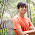Can you please write a prog on division using functions.

1.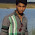HI pavan, for getting division of two numbers using function,
in the above program just change the following

c = division(a,b);

int division( int num1,int num2)
{
int result; /* defining variable, its scope lies within function */
result = num1 / num2 ; /*division of two numbers*/
return (result) ; /* returning result */
}

2.great explanation

3.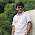#include
int div(int,int);
int main(void)
{
int a,b,result;
printf("\nenter the two integers:\n");
scanf("%d%d",&a,&b);
result=div(a,b);
printf("\nthe division of %d and %d is %d",a,b,result);
return 0;
}
int div(int p ,int q)
{
int c1;
if(q==0)
printf("\n UNDEFINED\n");
else
c1=p/q;
return (c1);

}

2.hi! can you please draw a flowchart of this program, i'll be very grateful, can't find any flowchart of functions anywhere

1.had anyone helped u in it Mr.Sharma?

3.4.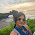addition of three num. using function. one should be in main nd others should be in function.. will u help me with this query?

1.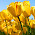#include
#include

int total (int, int);
int main()
{
int a, b, c, d, e;
d = 450;
printf("1st number is: %d\n",d);
printf("Now Enter other the two numbers:\n\n");
scanf("%d %d", &a, &b);
c = total(a, b);
e = c + d;
printf("Total of three Numbers is: %d\n",e);
getch();
return 0;
}

int total(int num1, int num2)

{
int result;
result = num1 + num2;
return result;
}

5.#include #include int sum(int x,int y); //function declaration void main() { int a,b,c; clrscr(); printf("Enter the two numbers:"); scanf("%d%d",&a,&b); c=sum(a,b); //function call printf(" \n the sum of two number is : %d ",c); getch(); } int sum(int x,int y) //function definition { int c; c=x / y; return c; }

6.can any one help mw with finding function prototype with flow charts?

7.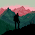Hey all you C lovers. Here are some programming exercises for beginners to practice and practice

Basic programming exercises in C
If else programming exercise to practice in C
Exercises on switch case in C
Exercises on conditional operator

Practice, Practice and Practice....

Happy coding ;)

8.I will like to know how to add to no. Which are declare in function n one function in main in c++

9.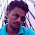#include
#include
int sum(int,int);
void main()
{
int a,b,c;
printf("\n Enter the two numbers : ");
scanf("%d %d",&a,&b);
c = sum(a,b);

printf("\n The sum of two numbers is : %d ",c);
getch();
}

int sum ( int num1,int num2)
{
int result;

return (result) ;

}

Sample Output: ( using GNU GCC Compiler with Code Blocks IDE )

Enter the two numbers: 10 15
The sum of two numbers is: 25

10.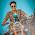This comment has been removed by the author.

11.pls someone solve it...
que-write a c program to create 2 single dimensional array size by store data into the 2nd array.display the data both the erray?

12.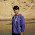add two numbers with arguments and without
return values .... plz help

1.Hi, We can also add two numbers using pointers in C.

firstNumberPointer = &firstNumber;
secondNumberPointer = &secondNumber;
sum = *firstNumberPointer + *secondNumberPointer;

13.This blog awesome and i learn a lot about programming from here.The best thing about this blog is that you doing from beginning to experts level.

Love from

14.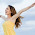Write function max(), this function find the maximum number between three numbers Write a program that uses this function to allow user enter numbers and print maximum number

15.#include
void subtraction();
void multiplication();
void division();
Int a,b,choice;
int main ()
Printf("value of a");
Scanf("%d",&a);
Printf("value of b");
Scanf("%d",&b);
printf("please type /n 1 for add/n 2/ for sub/n 3 for mul/n 4 for div/n");
scanf("%d",&choice);
if(choice==1)
{
}
if(choice==2)
{
void subtraction()
if(choice==2)
{
void multiplication()
}
if(choice==4)
{
void division()
}
return 0;
}
{
printf("sum of %d and %d is %d",a,b,(a+b));
}
void subtraction();
{
printf("sub of %d and %d is %d",a,b,(a-b));
}
void muptiplication();
{
printf("mul of %d and %d is %d",a,b,(a*b));
}
void division();
{
printf("div of %d and %d is %d",a,b,(a/b));
}

16.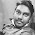please tell me how to print the result in main if function is void type

1.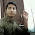Hi, In this case, you can use a global variable.

#include
int result; /*global variable*/
int main()
{
int a=10,b=20;
sum(a,b);
printf("\n The sum of two numbers is : %d ",result);
return 0;
}

sum(int num1,int num2)
{
result = num1 + num2 ; /*adding two numbers and storing result in global variable*/
}

17.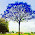I write same in code::block but it's not working

18.Everything worked....plz elaborate use of scanf and printf .....why %d is used....

1.%d is used as a format specifier, here is a samll example, go through this link http://cprogramsblog.blogspot.com/2012/07/c-program-for-showing-printf-format.html

19.Thanks for sharing this post. First Program in C Language .